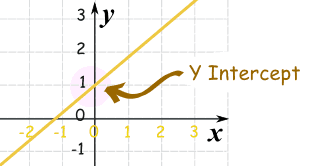# Slope Intercept Form

Standard

### y = mx + b

m = Slope (how steep the line is)

b = the Y Intercept (where the line crosses the Y axis)\### How do you find “m” and “b“?

• b is easy: just see where the line crosses the Y axis.
• m (the Slope) needs some calculation. Remember we like to write slope like a fraction.

## Example 1)The fastest and easiest thing to find first when looking at a graph is the y-intercept (b).

Here we see that the line crosses the y-axis at positive 1.

So, b = 1 .

Now to find the slope (m) we will use rise over run:

• Rise is how far up
• Run is how far along

In this example the rise is 2 and the run is 1.

So, m = 2/1 .

Now that I know m = 2/1 and b = 1 I can plug them into the equation for slope intercept form y = mx + b.

y = (2/1) x + 1

^^^^^ This is the equation of the line.

# Y – intercept

Standard

## Y Intercept

Where a straight line crosses the Y axis of a graph.

### Example:In the above diagram the line crosses the Y axis at 1.

So the Y intercept is equal to 1.

## Practice Problems

TEST YOUR SELF ON LINES HERE!!!!

# Interest

Standard

### Introduction to interest:

http://www.mathsisfun.com/money/interest.html

SIMPLE INTEREST

I = Prt

• I = interest  [\$]
• P = amount borrowed (called “Principal”)  [\$]
• r = interest rate   [%]
• t = time    [years]

COMPOUND INTEREST

A = P(1+r)^t

• A = actual amount owed (total debt)   [\$]
• P = amount borrowed (called “Principal”)    [\$]
• r = interest rate     [%]
• t = time    [years]

Information on compound interest:

http://www.mathsisfun.com/definitions/compound-interest.html

SIMPLE INTEREST PRACTICE PROBLEMS:

http://www.mathopolis.com/questions/q.php?id=1702&site=1&ref=/money/interest.html&qs=1702_1703_1704_1705_3749_3750_3751_1706_1707_1708

COMPOUND INTEREST PRACTICE PROBLEMS:

http://www.mathopolis.com/questions/q.php?id=2294&site=1&ref=/money/compound-interest.html&qs=2294_2295_2296_2297_2298_2299_2300_2301_2302_2303